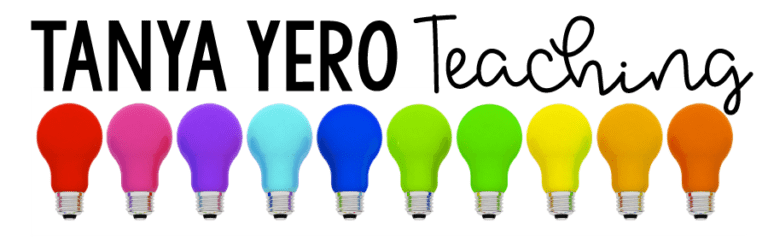Sale!

# 3rd Grade Guided Math YEARLONG BUNDLE Differentiated Work EASY PREP

\$28.95 \$22.00

Total Pages: 150+
File Size: 9 MB

## Description

Take the prep work out of guided math rotations and make life easier for yourself. POWER Math Reference Mats tailor instruction for your students with NO PREP on your behalf! Differentiate math with ease!

What are Guided Math Reference Mats?

POWER Math Reference Mats are designed to assist teachers during math rotations. Housed with specific target skills for each level in your class, POWER Math Reference Mats come with various problems for you to give to your students when working in small groups. Group A is for students who are in need of remediation, Group B is for students working on grade level, and Group C is for students who need enrichment. Each mat comes with target skills that are designed to match the needs of each student level in your class. There are questions/problems for each target skill which can be given to your students. Power Math Reference Mats require little materials: whiteboards, expo markers, and erasers or paper and pencils.

What domains are included?
✭Numbers and Operations in Base Ten
✭Fractions
✭Operations and Algebraic Thinking
✭Measurement and Data
✭Geometry

Standards & Topics Covered

Number and Operation in Base Ten
➥ 3.NB.1 – Place value concepts
➥ 3.NBT.2 – Adding & subtracting whole numbers
➥ 3.NBT.3 – Multiplying numbers

Operations & Algebraic Thinking
➥ 3.OA.1 – Interpreting products of whole numbers
➥ 3.OA.2 – Interpreting quotients of whole numbers
➥ 3.OA.3 – Use multiplication and division to solve word problems
➥ 3.OA.4 – Determining unknown numbers in a multiplication or division equation
➥ 3.OA.5 – Apply properties of operations to multiply and divide
➥ 3.OA.6 – Understand division as an unknown-factor problem.
➥ 3.OA.7 – Fluently multiply and divide within 100
➥ 3.OA.8 – Solve two-step word problems using the four operations.
➥ 3.OA.9 – Understanding patterns on a multiplication chart

Number and Operation – Fractions
➥ 3.NF.1 – Understanding fractions
➥ 3.NF.2 – Understanding fractions on number lines
➥ 3.NF.3 – Equivalent fractions and comparing fractions

Measurement and Data
➥ 3.MD.1 – Understanding time
➥ 3.MD.2 – measuring and understanding liquid volume and mass
➥ 3.MD.3 – Picture graphs
➥ 3.MD.4 – Measuring length and using line plots
➥ 3.MD.5 – Understanding area
➥ 3.MD.6 – Measuring area
➥ 3.MD.7 – Relate area to the operations of multiplication and addition.
➥ 3.MD.8 – Word problems with area and perimeter

Geometry
➥ 3.G.1 – Understanding and examining shapes
➥ 3.G.2 – Partition shapes into parts with equal areas.